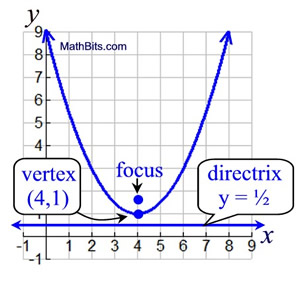Parabola Equation Practice Terms of Use    Contact Person: Donna Roberts1.
Find the focus and directrix of the parabola y = ¼ x2.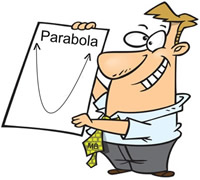Choose:
 focus: (0,2); directrix: y = -2 focus: (0,¼); directrix: y = -¼ focus: (0,1); directrix: y = -1 focus: (0,4); directrix: y = -4

2.
Write the equation of a parabola with a focus of (0,-2) and a directrix y = 2.Choose:
 y =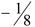x2 y2 = -4x y =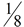x2 y2 = -8x

3.
Find the focus and directrix of the parabola y - 3 = -(x + 2)2.Choose:
 focus: (-2,3); directrix: y = -3 focus: (-2,3¼); directrix: y = 2¾ focus: (-2,2¾); directrix: y = 3¼ focus: (-2,3); directrix: y = 4

4.
Write the equation of a parabola with a vertex of (-1,4) and a directrix of x = 1.Choose:
 (x + 1)2 = 2(y - 4) (x - 1)2 = -2(y + 4) (y + 4)2 = 8(x - 1) (y - 4)2 = -8(x + 1)

5.
Given parabola x = (y + 5)2 + 2. What is the equation of its directrix?Choose:
 x = 2 x = 1¾ y = -5 y = 5¼

6.
Given the graph shown at the right, find the equation of the parabola. (You may assume that the vertex coordinates and the directrix equation have integer values.)
Choose:
 (x + 2)2 = 4(y - 4) (x + 2)2 = (y - 4) (x - 2)2 = 4(y + 4) (x - 2)2 = (y + 4)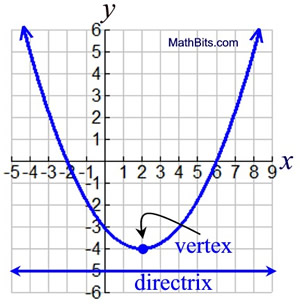7.
This NASA tracking antenna is a parabolic dish. Its receiver is placed at its focus 27 meters from the center of the dish (its vertex). For this problem, assume the dish is pointing upward and represent the vertex as (0,0). Write a parabolic equation that models the cross section of the antenna dish.
Choose:
 x2 = 27y x2 = 54y x2 = 108y y2= 54xPhoto courtesy of NASA.
This is a 70 meter tracking antenna (with a parabolic dish) weighing nearly 2,970 ton.

8.
A parabola has its vertex at the origin and focus at (0,4). Find the length of the segment that passes through the focus, is parallel to the directrix, and whose endpoints lie on the parabola.Choose:
 length = 8 units length = 16 units length = 32 inches length = 64 units

9.
Write the equation of a parabola with a focus of (-1,-2) and a directrix y = -4.Choose:
 (x + 1)2 = 4(y + 3) (x+ 1)2 = ¼(y + 3) (x - 1)2 = 4( y - 3) (x - 1)2 = ¼(y - 3)

 10. Which of the following parabolic equations could be used to represent the graph at the right? (Check all that apply, and hit SUBMIT!) x2 - 8x - 2y + 18 = 0 (y - 1)2 = 2(x - 4) y = ½(x - 4)2 + 1 x2 - 8x - 2y + 14 = 0 y = ½x2 2(y - 1) = (x - 4)2 (y - 1)2 = ½(x - 4)